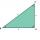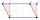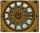# The perimeter of parallelogram problems

#### Number of problems found: 10

• Parallelogram perimeterThe ABC triangle with sides a = 5cm, b = 3cm, c = 40mm has the center of the sides of the K,L,M. How many cm have the KBLM parallelogram perimeter?
• ParallelogramThe parallelogram has sides lengths in the ratio 3: 4 and perimeter 2.8 meters. Determine the lengths of the sides.
• ParallelogramThe perimeter of the parallelogram is 417 cm. The length of one side is 1.7-times longer than the length of the shorter side. What is the length of sides of a parallelogram?
• The parallelogramThe parallelogram has one side 2 dm long, which is one-sixth of its circumference. How many cm does the other side of the parallelogram measure?
• ParallelogramSide a of parallelogram is twice longer than side b. Its circumference is 78 dm. Calculate the length of the sides of a parallelogram.
• ParallelogramCalculate the area and perimeter of a parallelogram whose two sides are long a=24 cm b=22 cm and height ha = 6 cm long.
• Rectangle - parallelogramIt is given a rectangle that is circumscribed by a circle with a radius of 5 cm. The short side of the rectangle measures 6 cm. Calculate the perimeter of a parallelogram ABCD whose vertices are the midpoints of the sides of the rectangle.
• ParallelogramCalculate the missing side of a parallelogram, if you know the perimeter o and one side: a) o = 7.2 cm; b = 1.8 cm b) o = 5.4 cm; a = 1.9 cm
• ParallelogramFind the perimeter of the parallelogram, where base a = 8 cm, height v = 3 cm, and angle alpha = 35° is the magnitude of the angle at vertex A.
• MidpointsTriangle ABC with sides a = 5 cm, b = 3 cm, c = 40mm has a midpoint of K, L, M. How many centimeters is long perimeter of parallelogram KBLM?

We apologize, but in this category are not a lot of examples.
Do you have an interesting mathematical word problem that you can't solve it? Submit a math problem, and we can try to solve it.

We will send a solution to your e-mail address. Solved examples are also published here. Please enter the e-mail correctly and check whether you don't have a full mailbox.

Please do not submit problems from current active competitions such as Mathematical Olympiad, correspondence seminars etc...

Perimeter - math word problems. Parallelogram Problems.